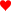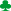# Poker Ratio Odds Chart

Odds Charts: Ratio Chart : Percentage Chart : Conversion Chart

This ratio poker odds chart highlights the ratio odds of completing your draw based on the number of outs you have at different points in a hand. The odds of completing your draw have been rounded to 1 decimal place in this ratio chart.

Look below the table for more information on how to use the ratio odds chart.

## Ratio odds chart.

Outs 1 Card To Come (flop) 1 Card To Come (turn) 2 Cards To Come (flop)
1 46.0 to 1 45.0 to 1 22.5 to 1
2 22.5 to 1 22.0 to 1 10.9 to1
3 14.7 to 1 14.3 to 1 7.0 to 1
4 (gutshot) 10.8 to 1 10.5 to 1 5.1 to 1
5 8.4 to 1 8.2 to 1 3.9 to 1
6 6.8 to 1 6.7 to 1 3.1 to 1
7 5.7 to 1 5.6 to 1 2.6 to 1
8 (straight) 4.9 to 1 4.8 to 1 2.2 to 1
9 (flush) 4.2 to 1 4.1 to 1 1.9 to 1
10 3.7 to 1 3.6 to 1 1.6 to 1
11 3.3 to 1 3.2 to 1 1.4 to 1
12 2.9 to 1 2.8 to 1 1.2 to 1
13 2.6 to 1 2.5 to 1 1.1 to 1
14 2.4 to 1 2.3 to 1 1.0 to 1
15 (s + f) 2.1 to 1 2.1 to 1 0.9 to 1
16 1.9 to 1 1.9 to 1 0.8 to 1
17 1.8 to 1 1.7 to 1 0.7 to 1
18 1.6 to 1 1.6 to 1 0.6 to 1
19 1.5 to 1 1.4 to 1 0.5 to 1
20 1.4 to 1 1.3 to 1 0.5 to 1
21 1.2 to 1 1.2 to 1 0.4 to 1
22 1.1 to 1 1.1 to 1 0.4 to 1

## Ratio table key.

• (flop) and (turn) - Indicates where you are in the hand when trying to find your odds.
• Gutshot - A straight draw with only one card able to complete it. e.g. 6-8 on a 5-9-Q board (only a 7 completes).
• Straight - A standard open-ended straight draw with more outs. e.g. 6-8 on a 5-7-Q board (4 and 9 complete).
• Flush - A hand where another card of the same suit is needed to complete the draw.
• s + f - Both an open ended straight draw and flush combined. e.g. 68on a 57Qboard.

## How to use the ratio odds chart.

1. Work out the number of outs you have (use the colours to help guide you).
2. Look up the ratio odds of completing your draw depending on whether you are on the flop or turn.

That's simple enough, but why are there 2 columns for percentage odds on the flop? The first 2 columns with 1 card to come are the odds that you should be using most frequently. These are the standard odds that assume we could potentially face another bet on the next betting round.

The last 2 cards to come column is for when you or your opponent are being placed all in on the flop. Therefore, because you do not expect to have to call another bet or raise on future betting rounds, you can now use these improved odds for seeing 2 cards instead of 1.

If you want to try working out the ratio odds for lots of different types of draws, have a look at my article on working out odds for uncommon draws in Texas Hold'em.

## How to turn a ratio in to a percentage.

Add the two numbers in the ratio together. Then divide 100 by that number.

So for example, if you have a flush draw on the turn, the odds of completing your draw are 4.1 to 1 (let's call it 4 to 1).

• 4 to 1 odds, so 4 + 1 = 5.
• 100 / 5 = 20%.

So if you have 4 to 1 odds, you have a 20% chance of completing your draw. Easy.

Note: Your ratio must be in the format of "X to 1" first before this method will work. So for example, you would need to turn 9 to 2 in to 4.5 to 1 first. Most ratios in poker are in the X to 1 format anyway, so it's not a big concern.

## Quick ratio odds chart example.

Lets say you are on the turn waiting for the river, and are holding a flush draw; you have 4.1 to 1 odds (roughly 4 to 1).

If you need to call \$40 when the pot is \$120 to see the next card you should fold, because these pot odds are 3 to 1 which makes the call unprofitable in the long run.

If the pot odds are smaller than the odds of completing your draw, you should fold.

For more information on working out ratio odds with drawing hands, see the pot odds article.

Go back to the poker odds charts.

Have You Not Heard Of
Deuces Cracked?“I quit my job and started playing poker back in February of 08',
after a month of losing over 50BI at micro stakes, I joined DC. I've
been living off poker ever since; if anything in this world could help
you become a winning player, this is it.”
- Malefiicus, DC Member

# Subscribe to thepokerbank

I'll send you an email if I add something new and interesting to the website.

Don't worry, it doesn't happen very often.